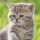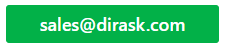Home
IT Knowledge
IT Career
Inspiration
Languages
EN

# Java - fastest way to negate number

6 points
Created by:Kate_C
19790

In Java it is possible to negate numbers in following ways:

1. `x = -x`
2. `x *= -1`
3. `x = ~x + 1`

## 1. Complexity analysis

1. `x = -x` operations:
2. make value negation
3. write value to memory
2. `x *= -1` (`x = x * (-1)`) operations:
2. make multiplication by `-1` operation
3. write value to memory
3. `x = ~x + 1` operations:
2. make bitwise not operation
3. make add `+1` operation
4. write value to memory

Notes:

• multiplication operations are slower than bitwise and subtract operations,
• in this case interpreter / compliler can make some optimisation,
• amount of operations affects on perfomrance,
• getting and setting variables from RAM memory can be slow.

The best way is always to make a benchmark test.

## 2. Benchmark test example

``````package com.dirask.examples;

public class Program {

public static class Result {
public String name;
public long dt;

public Result(String name, long dt) {
this.name = name;
this.dt = dt;
}
}

public static void main(String[] args) {
// configutarion

int testsCount = 1;
int testSize = 100000000;

// test

Result result1 = new Result("x = -x;", 0);
Result result2 = new Result("x *= -1;", 0);
Result result3 = new Result("x = ~x + 1;", 0);

long t1, t2;
long x = Math.round(1000 * Math.random());

for (int i = 0; i < testsCount; ++i) {

t1 = System.nanoTime();
for (int j = 0; j < testSize; ++j) {
x = -x;
}
t2 = System.nanoTime();
result1.dt += t2 - t1;

t1 = System.nanoTime();
for (int j = 0; j < testSize; ++j) {
x *= -1;
}
t2 = System.nanoTime();
result2.dt += t2 - t1;

t1 = System.nanoTime();
for (int j = 0; j < testSize; ++j) {
x = ~x + 1;
}
t2 = System.nanoTime();
result3.dt += t2 - t1;
}

// summary

Result[] results = { result1, result2, result3 };
Result max = results;

for (int i = 1; i < results.length; ++i) {
Result result = results[i];

if (result.dt > max.dt) {
max = result;
}
}

System.out.println("x=" + x);

for (int i = 0; i < results.length; ++i) {
Result result = results[i];

if(result == max) {
System.out.println(result.name
+ " // " + (result.dt / 1000000) + "ms");
} else {
double imprDifference = max.dt - result.dt; // improvement
double imprPercentage = 100 * imprDifference / max.dt;

String imprText = String.format("%.2f", imprPercentage);

System.out.println(result.name
+ " // " + (result.dt / 1000000) + "ms"
+ " -> " + imprText  + "% faster than " + max.name);
}
}
}
}``````

Output:

``````x=106
x = -x; // 10ms -> 88,90% faster than x = ~x + 1;
x *= -1; // 6ms -> 92,91% faster than x = ~x + 1;
x = ~x + 1; // 91ms``````

Note: above test has been made on PC:

• Notebook: Xiaomi Mi 13
• OS: Windows 10 x64
• JVM: Java Hotspot 64-Bit (1.8.0_201)
• CPU: Intel i5-6200u (6th generation)
• RAM: DDR4 8GB 2133 MHz
• SSD: SAMSUNG MZVLV256HCHP-00000

### 3.1 PC - CPU i5 4460

PC Specification:

• OS: Windows 10 x64
• JVM: Java Hotspot 64-Bit (1.8.0_221)
• CPU: Intel i5-4460 Processor (4th Generation)
• RAM: DDR3 16GB 1600MHz - Crucial Ballistix Sport CL9
• SSD: SAMSUNG SSD 840 EVO 500GB

Output:

``````// test 1
x=32
x = -x; // 5ms -> 92.34% faster than x = ~x + 1;
x *= -1; // 3ms -> 94.74% faster than x = ~x + 1;
x = ~x + 1; // 69ms

// test 2
x=434
x = -x; // 4ms -> 93.54% faster than x = ~x + 1;
x *= -1; // 3ms -> 95.26% faster than x = ~x + 1;
x = ~x + 1; // 70ms

// test 3
x=25
x = -x; // 5ms -> 92.43% faster than x = ~x + 1;
x *= -1; // 3ms -> 95.27% faster than x = ~x + 1;
x = ~x + 1; // 67ms

# summary

x = -x; // 0 points
x *= -1; // 3 points
x = ~x + 1; // 0 point``````